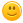193 views
Omg I need help on multiplication I'm starting division help meeeeeeeeeeeeeeeeeeeee!!!!!!!
commented Apr 24, 2020 by cookie monster

get a multiplication chart or get extra math it's a really fun math site!commented Nov 19, 2020 by Bugaboo

answered Oct 11, 2020 by #softballstar25
answered Oct 1, 2020 by Virgo
Umm... You could just get flash cards?  I started off skip counting and that helped.
answered May 5, 2020 by (32,600 points)
Multiplication is just repeated addition, if that helps at all. Division is pretty easy once you memorize Your multiplication tables, quarantine is a great time to start memorizing. Division is just the flip side of multiplication, if that makes sense.
answered May 5, 2020 by (15,790 points)

If you know your multiplication then you can easily do division. Here's some 2nd grade math.

If you know your times tables for 4, like 4x4 then you know 20 divided by 4. If you don't, then add on

4+4=8

8+4=12

12+4=16

16+4=20

Easy peasy 3rd grade stuff! 20 divided by 4? Just add on 4 with your fingers until you get to 20! The number on your hands is the answer!! If you have double digit division then do this

20

x12

240

240 divided by 12.  Just multiply the numbers on the tens side and then carry them to the other side, I'm not smart enough to explain the other carrying stuff.

answered Feb 12, 2020 by (374,140 points)

i am so superior i am in seventh grade amiright im just so superior then your children

that's not the point lol, point is i can help!